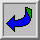### The completeness theorem for first order logic

G—del was the first to prove this theorem (in his doctoral thesis). It's sometimes referred to as "G—del's completeness theorem", chiefly in order to confuse people.

The completeness theorem for so-called first order logic is a very basic result in logic, used all the time. The formalized mathematical theories T usually discussed in connection with G—del's theorem - such as axiomatic set theory ZFC and formal arithmetic PA - are subject both to the incompleteness theorem and to the completeness theorem. There is no conflict here, for "complete" means different things in the two theorems.

That T is incomplete in the sense of the incompleteness theorem means that there is some statement A in the language of T such that neither A nor its negation can be proved in T.

What is complete in the sense of the completeness theorem is not T, but first order logic itself. That first order logic is complete means that every statement A in the language of T which is true in every model of the theory T is provable in T. Here a "model of T" is an interpretation (in a mathematically defined sense) of the basic concepts of T on which all the axioms of T are true.

Thus, in particular, since the G—del sentence G is undecidable in T, there is a model of T in which G is false, and there is another model in which G is true. Since (for the usual theories T) the sentence G is true as ordinarily interpreted, a model in which G is false will be what is called a non-standard model. Such non-standard models are not usually of interest in mathematics, with one exception: they are explicitly introduced and systematically exploited in the field of mathematics known as non-standard analysis.

It is confusing that the term "complete" is used in different senses in the incompleteness theorem and in the completeness theorem, and this confusion is often reflected on the net in such comments as

Godel is also responsible for proving (1930) that first order predicate logic *is* complete. The incompleteness proofs apply to formal systems strong enough to represent the truths of arithmatic.